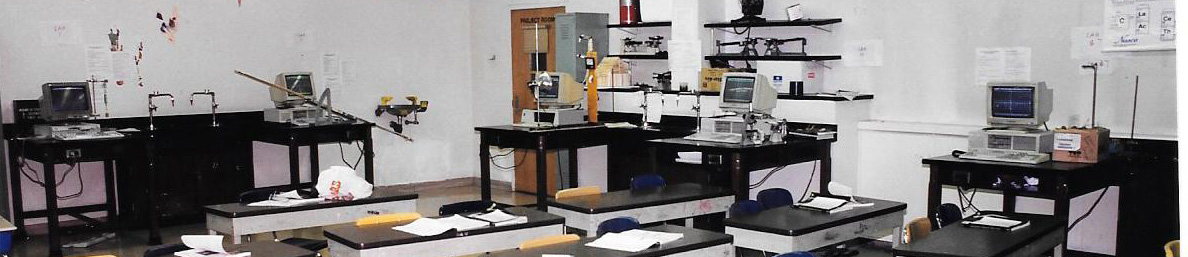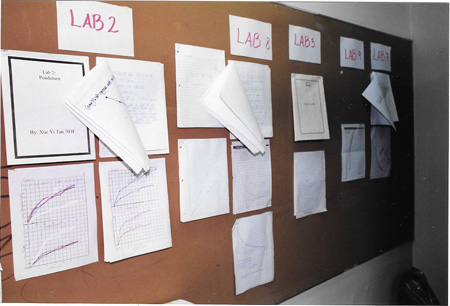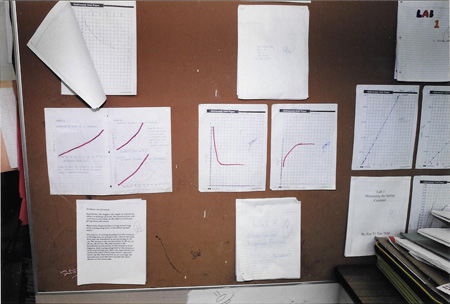# Mathematical Relationships in Science-- A STEM Laboratory ExerciseA Partial View of the Science Classroom showing 5 laboratory setups: (l-r) The Spring Constant, Acceleration Lab, LIght Inensity, Attraction and Repulsion Lab and Damping Motion

Ideally, most teachers  would like to schedule laboratory experiments once a week immediately following the time when the relevant material has been presented in class.  This approach has a particular appeal because students can immediately apply the principles that they have just learned from classroom lessons and the text.  With lack of sufficient funding and a reduced number  of lab specialists  in schools, many teachers have turned more toward classroom demonstrations.

The Rotating Laboratory Approach:

Using this approach the teacher only has to prepare one set-up for each experiment. It not only saves time for teachers, but allows students  to work with more expensive, elaborate, and interesting equipment . Each individual is assigned to a laboratory group of  three  or four students which remain in the same group throughout the laboratory sequence.  Each group has a unique schedule so that no two groups will require the same station simultaneously. Each week the group will rotate from one lab station to the next.  All students are given a manual  which contains all the lab assignments and the rules that need to be followed while doing the experiments. It is necessary for the teacher to demonstrate each of the labs to the whole class prior to beginning the cycle.   Mathematical relationships in Science The following labs were designed for use in middle school physical science classes  beginning in 1990. The photos shown here were taken in 1998  (as visible in the design of computers at each station. The classroom was located in the lower east side of Manhattan and had 10 lab stations which surrounded  the classroom.

The Mathematical relationships Module consists of 12  laboratory exercises.

Lab 1:    The Spring Constant  -- Problem:  What is the relationship between how much a spring stretches and the force pulling on the spring?

Lab 2:    The Pendulum  -- Problem: What is the relationship between the period of a pendulum and the length of the string of  the pendulum?

Lab 3:   Mass, Volume and Density--   Problem: What is the relationship between the mass of a ball and its volume assuming a constant density?

Lab 4:   Light Intensity-- Problem: What is the relationship between the intensity of a beam of light and the distance from a light source?

Lab 5:   Acceleration --  Problem: What is a the relationship between how the distance travels and the time in travel for an accelerating object?

Lab 6:   Polarization --  Problem:  What is the relationship between how much light passes through a Polaroid filter and the angle the filter is rotated?

Lab  7:   Ohms Law --  Problem:  What is the relationship between current, voltage when there is a constant resistance in an electric circuit.

Lab 8:   Radioactive Decay -- Problem: What is the relationship between the decay of radioactive material and the time allowed for the decay?

Lab 9:  Water Pressure -- Problem: What is the relationship between water pressure and depth of water?

Lab 10:  Attractive and Repulsive Forces-- Problem: What is the relationship between the distance between two magnets  and the force between them?

Lab 11:  Damping Motion --Problem: What is the relationship between the height a ball bounces and the number of times it has bounced?

Lab 12: Buoyancy Lab Problem: What is the relationship between the volume of a boat and the weight it can hold?

### Display of Student Work

Display of student work after the first lab allows students to compare their data with other students and get critical feedback on how to properly write up the final lab report.What is a mathematical relationship and what are the different types of mathematical relationships that apply to the laboratory exercises in the following activities.

#### Labs

Lab 1:    The Spring Constant  -- Problem:  What is the relationship between how much a spring stretches and the force pulling on the spring?

Lab 2:    The Pendulum  --Problem: What is the relationship between the period of a pendulum and the length of the string of  the pendulum?

Lab 3:   Mass, Volume and Density--   Problem: What is the relationship between the mass of a ball and its volume assuming a constant density?

Lab 4:   Light Intensity--  Problem: What is the relationship between the intensity of a beam of light and the distance from a light source?

Lab 5:   Acceleration--  Problem: What is a the relationship between how the distance travels and the time in travel for an accelerating object?

Lab 6:   Polarization --  Problem:  What is the relationship between how much light passes through a Polaroid filter and the angle the filter is rotated?

Lab  7:   Ohms Law--  Problem:  What is the relationship between current, voltage when there is a constant resistance in an electric circuit.

Lab 8:   Radioactive Decay-- Problem: What is the relationship between the decay of radioactive material and the time allowed for the decay?

Lab 9: Water Pressure-- Problem: What is the relationship between water pressure and depth of water?

Lab 10: Attractive and Repulsive Forces--   Problem: What is the relationship between the distance between two magnets  and the force between them?

Lab 11: Damping Motion--  Problem: What is the relationship between the height a ball bounces and the number of times it has bounced?

Lab 12: Buoyancy - Problem: What is the relationship between the volume of a boat and the weight it can hold?